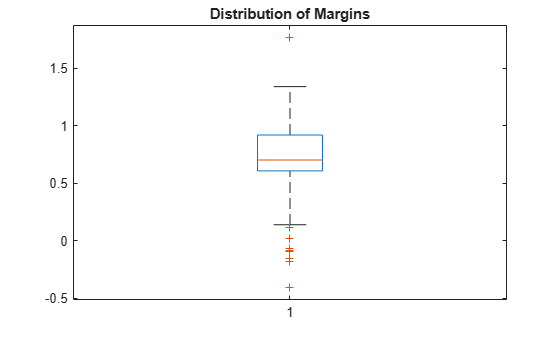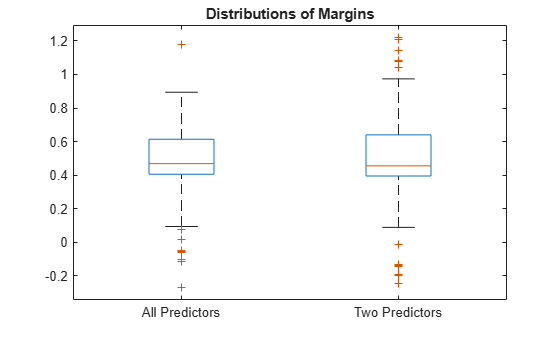# kfoldMargin

Classification margins for cross-validated ECOC model

## Syntax

``margin = kfoldMargin(CVMdl)``
``margin = kfoldMargin(CVMdl,Name,Value)``

## Description

example

````margin = kfoldMargin(CVMdl)` returns classification margins obtained by the cross-validated ECOC model (`ClassificationPartitionedECOC`) `CVMdl`. For every fold, `kfoldMargin` computes classification margins for validation-fold observations using an ECOC model trained on training-fold observations. `CVMdl.X` contains both sets of observations.```

example

````margin = kfoldMargin(CVMdl,Name,Value)` returns classification margins with additional options specified by one or more name-value pair arguments. For example, specify the binary learner loss function, decoding scheme, or verbosity level.```

## Examples

collapse all

Load Fisher's iris data set. Specify the predictor data `X`, the response data `Y`, and the order of the classes in `Y`.

```load fisheriris X = meas; Y = categorical(species); classOrder = unique(Y); rng(1); % For reproducibility```

Train and cross-validate an ECOC model using support vector machine (SVM) binary classifiers. Standardize the predictor data using an SVM template, and specify the class order.

```t = templateSVM('Standardize',1); CVMdl = fitcecoc(X,Y,'CrossVal','on','Learners',t,'ClassNames',classOrder);```

`CVMdl` is a `ClassificationPartitionedECOC` model. By default, the software implements 10-fold cross-validation. You can specify a different number of folds using the `'KFold'` name-value pair argument.

Estimate the margins for validation-fold observations. Display the distribution of the margins using a box plot.

```margin = kfoldMargin(CVMdl); boxplot(margin) title('Distribution of Margins')```One way to perform feature selection is to compare cross-validation margins from multiple models. Based solely on this criterion, the classifier with the greatest margins is the best classifier.

Load Fisher's iris data set. Specify the predictor data `X`, the response data `Y`, and the order of the classes in `Y`.

```load fisheriris X = meas; Y = categorical(species); classOrder = unique(Y); % Class order rng(1); % For reproducibility```

Define the following two data sets.

• `fullX` contains all the predictors.

• `partX` contains the petal dimensions.

```fullX = X; partX = X(:,3:4);```

For each predictor set, train and cross-validate an ECOC model using SVM binary classifiers. Standardize the predictors using an SVM template, and specify the class order.

```t = templateSVM('Standardize',1); CVMdl = fitcecoc(fullX,Y,'CrossVal','on','Learners',t,... 'ClassNames',classOrder); PCVMdl = fitcecoc(partX,Y,'CrossVal','on','Learners',t,... 'ClassNames',classOrder);```

`CVMdl` and `PCVMdl` are `ClassificationPartitionedECOC` models. By default, the software implements 10-fold cross-validation.

Estimate the margins for each classifier. Use loss-based decoding for aggregating the binary learner results. For each model, display the distribution of the margins using a boxplot.

```fullMargins = kfoldMargin(CVMdl,'Decoding','lossbased'); partMargins = kfoldMargin(PCVMdl,'Decoding','lossbased'); boxplot([fullMargins partMargins],'Labels',{'All Predictors','Two Predictors'}) title('Distributions of Margins')```The margin distributions are approximately the same.

## Input Arguments

collapse all

Cross-validated ECOC model, specified as a `ClassificationPartitionedECOC` model. You can create a `ClassificationPartitionedECOC` model in two ways:

### Name-Value Arguments

Specify optional pairs of arguments as `Name1=Value1,...,NameN=ValueN`, where `Name` is the argument name and `Value` is the corresponding value. Name-value arguments must appear after other arguments, but the order of the pairs does not matter.

Before R2021a, use commas to separate each name and value, and enclose `Name` in quotes.

Example: `kfoldMargin(CVMdl,'Verbose',1)` specifies to display diagnostic messages in the Command Window.

Binary learner loss function, specified as the comma-separated pair consisting of `'BinaryLoss'` and a built-in loss function name or function handle.

• This table describes the built-in functions, where yj is the class label for a particular binary learner (in the set {–1,1,0}), sj is the score for observation j, and g(yj,sj) is the binary loss formula.

ValueDescriptionScore Domaing(yj,sj)
`'binodeviance'`Binomial deviance(–∞,∞)log[1 + exp(–2yjsj)]/[2log(2)]
`'exponential'`Exponential(–∞,∞)exp(–yjsj)/2
`'hamming'`Hamming[0,1] or (–∞,∞)[1 – sign(yjsj)]/2
`'hinge'`Hinge(–∞,∞)max(0,1 – yjsj)/2
`'linear'`Linear(–∞,∞)(1 – yjsj)/2
`'logit'`Logistic(–∞,∞)log[1 + exp(–yjsj)]/[2log(2)]
`'quadratic'`Quadratic[0,1][1 – yj(2sj – 1)]2/2

The software normalizes binary losses so that the loss is 0.5 when yj = 0. Also, the software calculates the mean binary loss for each class .

• For a custom binary loss function, for example `customFunction`, specify its function handle `'BinaryLoss',@customFunction`.

`customFunction` has this form:

`bLoss = customFunction(M,s)`

• `M` is the K-by-B coding matrix stored in `Mdl.CodingMatrix`.

• `s` is the 1-by-B row vector of classification scores.

• `bLoss` is the classification loss. This scalar aggregates the binary losses for every learner in a particular class. For example, you can use the mean binary loss to aggregate the loss over the learners for each class.

• K is the number of classes.

• B is the number of binary learners.

For an example of passing a custom binary loss function, see Predict Test-Sample Labels of ECOC Model Using Custom Binary Loss Function.

This table identifies the default `BinaryLoss` value, which depends on the score ranges returned by the binary learners.

AssumptionDefault Value

All binary learners are any of the following:

• Classification decision trees

• Discriminant analysis models

• k-nearest neighbor models

• Naive Bayes models

`'quadratic'`
All binary learners are SVMs.`'hinge'`
All binary learners are ensembles trained by `AdaboostM1` or `GentleBoost`.`'exponential'`
All binary learners are ensembles trained by `LogitBoost`.`'binodeviance'`
You specify to predict class posterior probabilities by setting `'FitPosterior',true` in `fitcecoc`.`'quadratic'`
Binary learners are heterogeneous and use different loss functions.`'hamming'`

To check the default value, use dot notation to display the `BinaryLoss` property of the trained model at the command line.

Example: `'BinaryLoss','binodeviance'`

Data Types: `char` | `string` | `function_handle`

Decoding scheme that aggregates the binary losses, specified as the comma-separated pair consisting of `'Decoding'` and `'lossweighted'` or `'lossbased'`. For more information, see Binary Loss.

Example: `'Decoding','lossbased'`

Estimation options, specified as the comma-separated pair consisting of `'Options'` and a structure array returned by `statset`.

To invoke parallel computing:

• You need a Parallel Computing Toolbox™ license.

• Specify `'Options',statset('UseParallel',true)`.

Verbosity level, specified as the comma-separated pair consisting of `'Verbose'` and `0` or `1`. `Verbose` controls the number of diagnostic messages that the software displays in the Command Window.

If `Verbose` is `0`, then the software does not display diagnostic messages. Otherwise, the software displays diagnostic messages.

Example: `'Verbose',1`

Data Types: `single` | `double`

## Output Arguments

collapse all

Classification margins, returned as a numeric vector. `margin` is an n-by-1 vector, where each row is the margin of the corresponding observation and n is the number of observations (`size(CVMdl.X,1)`).

collapse all

### Classification Margin

The classification margin is, for each observation, the difference between the negative loss for the true class and the maximal negative loss among the false classes. If the margins are on the same scale, then they serve as a classification confidence measure. Among multiple classifiers, those that yield greater margins are better.

### Binary Loss

The binary loss is a function of the class and classification score that determines how well a binary learner classifies an observation into the class. The decoding scheme of an ECOC model specifies how the software aggregates the binary losses and determines the predicted class for each observation.

Assume the following:

• mkj is element (k,j) of the coding design matrix M—that is, the code corresponding to class k of binary learner j. M is a K-by-B matrix, where K is the number of classes, and B is the number of binary learners.

• sj is the score of binary learner j for an observation.

• g is the binary loss function.

• $\stackrel{^}{k}$ is the predicted class for the observation.

The software supports two decoding schemes:

• Loss-based decoding  (`Decoding` is `'lossbased'`) — The predicted class of an observation corresponds to the class that produces the minimum average of the binary losses over all binary learners.

`$\stackrel{^}{k}=\underset{k}{\text{argmin}}\frac{1}{B}\sum _{j=1}^{B}|{m}_{kj}|g\left({m}_{kj},{s}_{j}\right).$`

• Loss-weighted decoding  (`Decoding` is `'lossweighted'`) — The predicted class of an observation corresponds to the class that produces the minimum average of the binary losses over the binary learners for the corresponding class.

`$\stackrel{^}{k}=\underset{k}{\text{argmin}}\frac{\sum _{j=1}^{B}|{m}_{kj}|g\left({m}_{kj},{s}_{j}\right)}{\sum _{j=1}^{B}|{m}_{kj}|}.$`

The denominator corresponds to the number of binary learners for class k.  suggests that loss-weighted decoding improves classification accuracy by keeping loss values for all classes in the same dynamic range.

The `predict`, `resubPredict`, and `kfoldPredict` functions return the negated value of the objective function of `argmin` as the second output argument (`NegLoss`) for each observation and class.

This table summarizes the supported binary loss functions, where yj is a class label for a particular binary learner (in the set {–1,1,0}), sj is the score for observation j, and g(yj,sj) is the binary loss function.

ValueDescriptionScore Domaing(yj,sj)
`"binodeviance"`Binomial deviance(–∞,∞)log[1 + exp(–2yjsj)]/[2log(2)]
`"exponential"`Exponential(–∞,∞)exp(–yjsj)/2
`"hamming"`Hamming[0,1] or (–∞,∞)[1 – sign(yjsj)]/2
`"hinge"`Hinge(–∞,∞)max(0,1 – yjsj)/2
`"linear"`Linear(–∞,∞)(1 – yjsj)/2
`"logit"`Logistic(–∞,∞)log[1 + exp(–yjsj)]/[2log(2)]
`"quadratic"`Quadratic[0,1][1 – yj(2sj – 1)]2/2

The software normalizes binary losses so that the loss is 0.5 when yj = 0, and aggregates using the average of the binary learners .

Do not confuse the binary loss with the overall classification loss (specified by the `LossFun` name-value argument of the `kfoldLoss` and `kfoldPredict` object functions), which measures how well an ECOC classifier performs as a whole.

 Allwein, E., R. Schapire, and Y. Singer. “Reducing multiclass to binary: A unifying approach for margin classiﬁers.” Journal of Machine Learning Research. Vol. 1, 2000, pp. 113–141.

 Escalera, S., O. Pujol, and P. Radeva. “Separability of ternary codes for sparse designs of error-correcting output codes.” Pattern Recog. Lett., Vol. 30, Issue 3, 2009, pp. 285–297.

 Escalera, S., O. Pujol, and P. Radeva. “On the decoding process in ternary error-correcting output codes.” IEEE Transactions on Pattern Analysis and Machine Intelligence. Vol. 32, Issue 7, 2010, pp. 120–134.